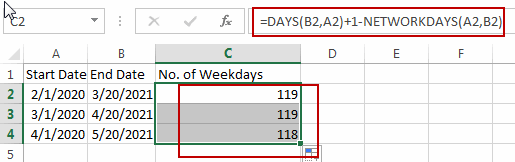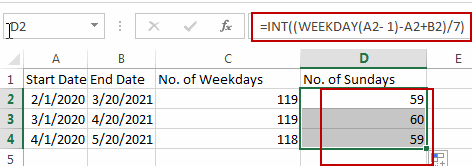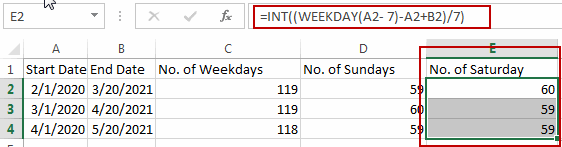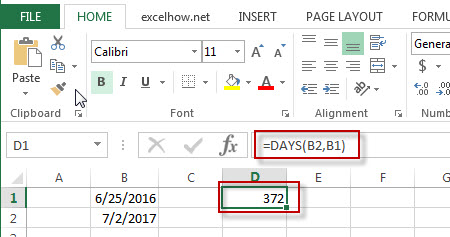## Calculate Days Open in Excel

If you want to know how to Calculate days in Excel, there are some formulas that you can use to do so. For example, you can use the DAYS function in Excel to find the number of days between two dates. However, if you want to find the number of days a ticket has been opened, you can use the if function.

This function calculates the number of days between two dates. It requires the date to be in an Excel-compatible format. Using the IF function, you can also specify the year, month, and day.

## 1. Calculate the Number of Days a Ticket Has Been Opened in Excel

It is important to track the time it takes to resolve support tickets whenever you are dealing with them. You can use Excel’s built-in function to calculate the backlog to do this. When your team members work long hours, the number of tickets they must resolve each day can increase significantly. In order to calculate the backlog, you first need to know the average resolution time of tickets. Once you have the average resolution time, you can subtract the open date from the current date to calculate the days a ticket has been open.

To calculate how many days a ticket has been open, you must first determine the date when the ticket was authored. You can also find out the number of days between a ticket’s date of issuance and its closing date by adding up the days between the two dates. Here’s an example. The ticket was created on April 18, 2022, and is still open. You can also calculate the number of days the ticket has been open using Excel. The formula is easy to use and makes analyzing your data easier.

``=IF(ISBLANK(D5),TODAY()-C5,D5-C5)``

If you want to calculate the number of days the spreadsheet has been open, you can use the IF function. In this case, you will need to input a date in a format that Excel understands.

## 2. Conclusion

You can use the IF function to Calculate the number of days a ticket remains open. You can enter the values in the formula, and a calculation will display the number of days that have passed since it was opened. The result of this calculation will be the number of days between the original ticket creation date and the date it was last reopened. By just putting in the values in the manner mentioned above, you can calculate the days opened!

## 3. Related Functions

• Excel ISBLANK function
The Excel ISBLANK function returns TRUE if the value is blank or null.The syntax of the ISBLANK function is as below:= ISBLANK (value)…
• Excel IF function
The Excel IF function perform a logical test to return one value if the condition is TRUE and return another value if the condition is FALSE. The IF function is a build-in function in Microsoft Excel and it is categorized as a Logical Function.The syntax of the IF function is as below:= IF (condition, [true_value], [false_value])….
• Excel TODAY function
The Excel TODAY function returns the serial number of the current date. So you can get the current system date from the TODAY function. The syntax of the TODAY function is as below:=TODAY()…
• Excel DAYS function
The Excel DAYS function returns the number of days between two dates.The syntax of the DAYS function is as below:= DAYS (end_date,start_date)…

## How to Calculate Number of Weekends between Two Dates in Excel

This post will guide you how to count the number of weekends between two given dates in Excel. How to find out how many weekends are between two dates in Excel. How to count the number of Sundays between two dates with a formula in Excel.

## Calculate the Number of Weekdays between Two Dates

Assuming that you have a list of data in range A1:B4, in which contain start dates and end dates. And you want to calculate the number of weekend days between start date and end date. How to do it. You can use a formula based on the NETWORKDAYS function and the DAYs function to calculate the number of weekends between two given dates.

Here is the formula that it will count the total number of weekend days between two given dates:

`=DAYS(B2,A2)+1-NETWORKDAYS(A2,B2)`

Type this formula into cell C2, and press Enter key on your keyboard, and then copy this formula from cell C2 to range C3:C4 to apply this formula.Let’s see how this formula works:

The NETWORKDAYS function try to calculate the number of working days (weekdays) between two dates, and the DAYS function will count the number of days between two dates. So you can use days number to subtract working days, then you will get the number of weekends days.

## Calculate the Number of Sundays between Two Dates

If you only want to count the number of Sundays between two given dates in Excel, you can use an Excel formula based on the INT function and the WEEKDAY function. Like this:

`=INT((WEEKDAY(B1- 1)-A2+B2)/7)`

Type this formula into cell D2, and press Enter key on your keyboard.Note: if you want to count the number of other week numbers (1-7, 1 is Sunday, 2 is Monday, 3 is Tuesday, 4 is Wednesday, 5 is Thursday, 6 is Friday, 7 is Saturday ) between two dates, such as: Saturday, you just need to replace the number 1 with 7 in the above formula. Like this:

`=INT((WEEKDAY(B1- 7)-A2+B2)/7)`### Related Functions

• Excel INT function
The Excel INT function returns the integer portion of a given number. And it will rounds a given number down to the nearest integer.The syntax of the INT function is as below:= INT (number)…
• Excel WEEKDAY function
The Excel WEEKDAY function returns a integer value representing the day fo the week for a given Excel date and the value is range from 1 to 7.The syntax of the WEEKDAY function is as below:=WEEKDAY (serial_number,[return_type])…
• Excel DAYS function
The Excel DAYS function returns the number of days between two dates.The syntax of the DAYS function is as below:= DAYS (end_date,start_date)…

## Excel Days Function

This post will guide you how to use Excel DAYS function with syntax and examples in Microsoft excel.

### Description

The Excel DAYS function returns the number of days between two dates.

The DAYS function is a build-in function in Microsoft Excel and it is categorized as a DATE and TIME Function.

The DAYS function is available in Excel 2016, Excel 2013.

### Syntax

The syntax of the DAYS function is as below:

= DAYS (end_date,start_date)

### Example

The below examples will show you how to use Excel DAYS Function to return the number of days between end_date and start_date.

#1 =DAYS (B2,B1)Note: the above excel formula retruns the number of days (372) between the end date (7/2/2017) and start date (6/25/2016).